# ScriptIQ for the non-developer

## Introduction

This tutorial provides a variety of examples of using ScriptIQ to create custom indicators.

The ScriptIQ scripting language is based on CoffeeScript. ScriptIQ simplifies development of custom indicators by providing a library of functions known as "descriptors" and "built-ins." Descriptors define (or describe) aspects of your custom indicators. Built-ins contain the logic and calculations for various technical indicators, such as moving averages. See the ScriptIQ API documentation for a complete list of descriptors and built-ins.

## ScriptIQ editor

Open the ScriptIQ editor. In technical-analysis-chart.html (see http://demo.chartiq.com), open the Studies menu, then select New Script in the SCRIPTIQ section of the menu.

## Plot of closing price

Let's start with a simple series that plots the "Close" values of the current dataset. Copy the following script into the ScriptIQ editor window, and then run the script. Inline comments, lines starting with #, explain what is going on in the code.

``````# Define the study and give it a name.
study("Closing Price")

# Define a study descriptor input.
field = input("Field", "Close")

# Draw the "Close" values and color the line chart orange.
plot(field, color: "orange")
``````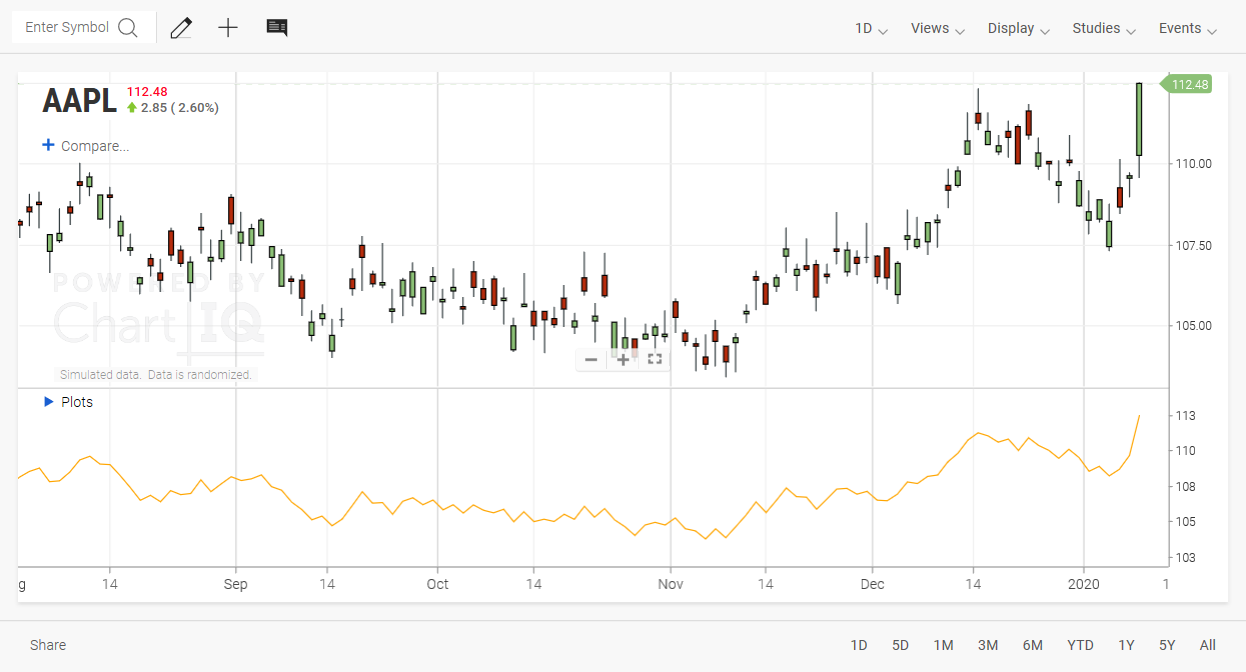Figure. Plot of closing price.

Your chart should now have a study panel containing the "Close" values from the dataset plotted as a line.

Note: When defining the field input, it is important to follow the naming scheme defined by your dataset. By default, the scheme is an OHLC format, and identifiers are case sensitive. For example:

``````Ask: 86.73
atr: 1.118418395205013
Bid: 86.55
BidHeatmap: Array
BidL2: Array
BidSize: 24
cache: Object
candleWidth: null
Close: 86.64
Date: "20200130000000000"
DT: [date] Thu Jan 30 2020 00:00:00 GMT-0500 (Eastern Standard Time)
High: 88.14
hl/2: 87.39
hlc/3: 87.14
hlcc/4: 87.015
iqPrevClose: 87.08
LastSize: 20
LastTime: [date] Thu Jan 30 2020 09:37:42 GMT-0500 (Eastern Standard Time)
Low: 86.64
ohlc/4: 87.30999999999999
Open: 87.82
ratio: 1
tick: 558
trueRange: 1.5
Volume: 40172672
``````

## Simple moving average of closing price

Now, let's make our indicator a little more interesting by creating a simple moving average of the closing price.

``````# Define the study and overlay it on the chart.
study("Closing Price SMA", overlay: true)
field = input("Field", "Close")

# Define the Period input for the study.
period = input("Period", 50)

# Use the sma built-in that calculates a simple moving average.
simple = sma(field, period)

plot(simple, color: "orange")
``````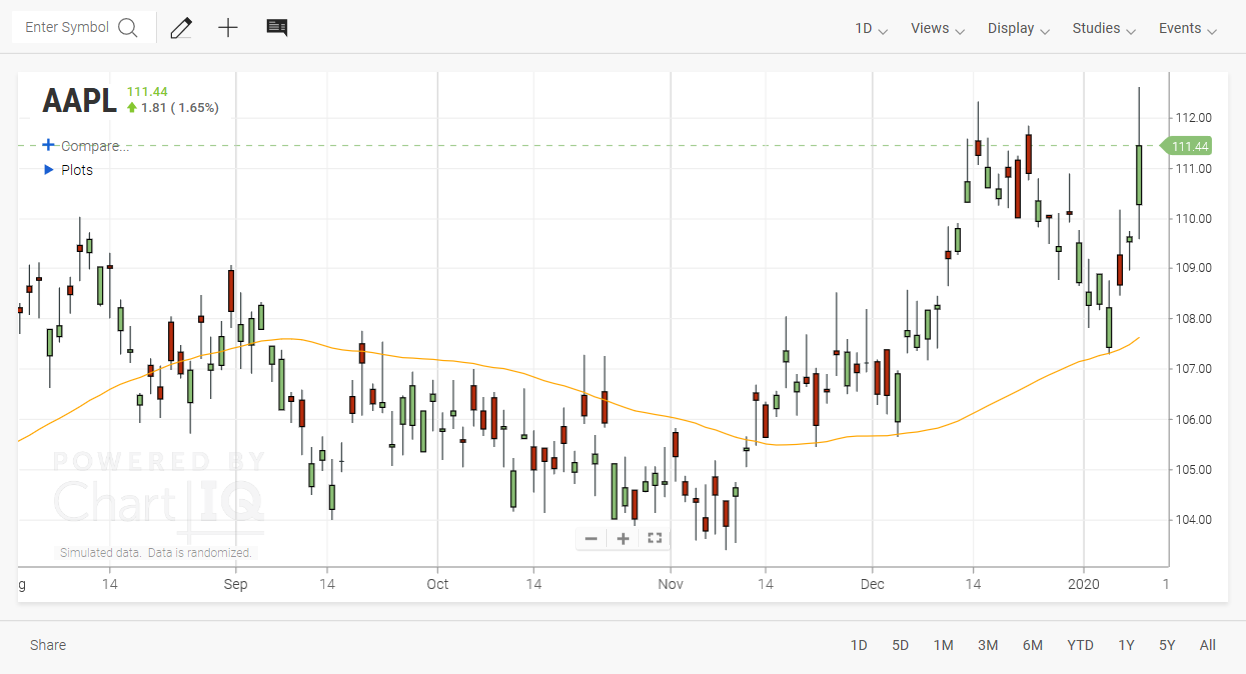Figure. Simple moving average of closing price.

The `sma` built-in provided by the ScriptIQ API provides a simple moving average calculation. Replace `sma` with `ema` (Exponential), `tma` (Triangular), or any of the other moving average built-ins described in the built-ins API documentation.

## Custom simple moving average of closing price

If the "sma" calculation in the previous example is not to your liking, you can rewrite the calculation with ScriptIQ.

``````study("Custom SMA")
field = input("Field", "Close")
period = input("Period", 50)
offset = input("Offset", 10)

# CoffeeScript function definition.
sum = (length) ->
total = 0
while length
value=dataset(field, -(--length))  # Use the dataset built-in. Start at the last data point and work backwards.
return null if not value?
total += value
total

# Call the defined sum function.
total = sum(period)
if total?
average = total / period  # Calculate the simple moving average.
plot(average, color: "orange")
``````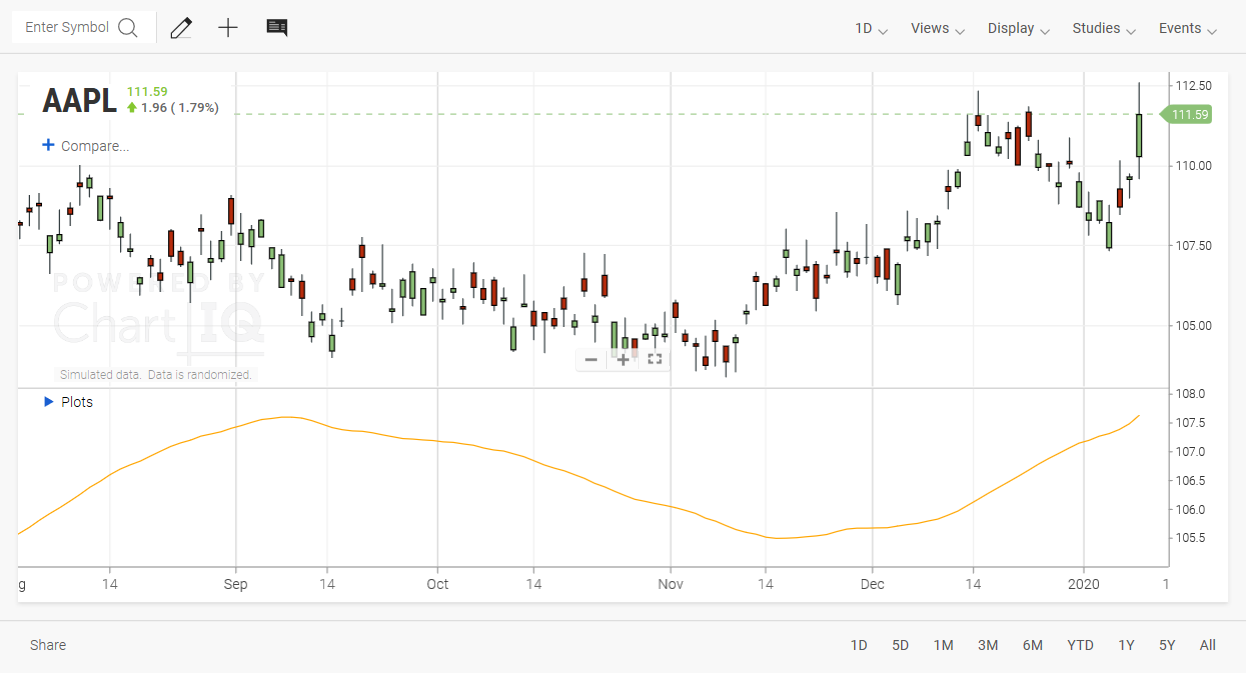Figure. Custom simple moving average of closing price.

## Simple MACD

Here's a simplification of the ChartIQ MACD study.

``````study("Simple MACD")
field = input("Field", "Close")
fast = input("Fast Period", 12)
slow = input("Slow Period", 26)
signal = input("Signal Period", 9)
fastMA = ema(field, fast)
slowMA = ema(field, slow)
macd = fastMA - slowMA
signalMA = sma(macd, signal)
plot(macd, color: "blue", display:"MACD")
plot(signalMA, color: "orange")
``````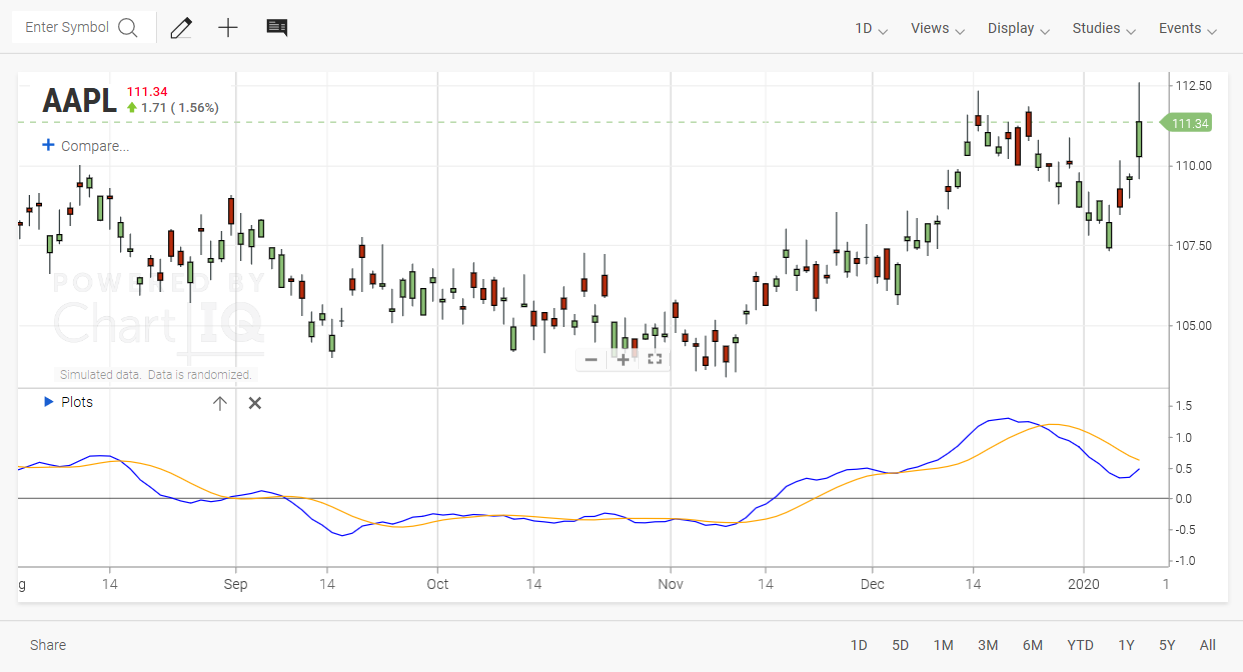Figure. Simple MACD.

## MACD with histogram and filled channels

ScriptIQ can plot more than just lines. This example uses the `histogram` and `fill` built-ins.

``````study("MACD Advanced")

field = input("Field", "Close")
fast = input("Fast MA Period", 12)
slow = input("Slow MA Period", 26)
period = input("Signal Period", 9)

fastMA = ema(field, fast)
slowMA = ema(field, slow)

macd = fastMA - slowMA
signal = ma(macd, period)

macdPlot = plot(macd, color: "blue")
signalPlot = plot(signal, color: "orange")

# Fill the space between the MACD line and the signal line.
fill(macdPlot, signalPlot, color: "#ffff00", opacity: 0.5)

# Create a histogram that represents the MACD.
histogram(macd - signal, colorIncreasing: "#00ffff", colorDecreasing: "#ff00ff", opacity: 0.9) if signal?
``````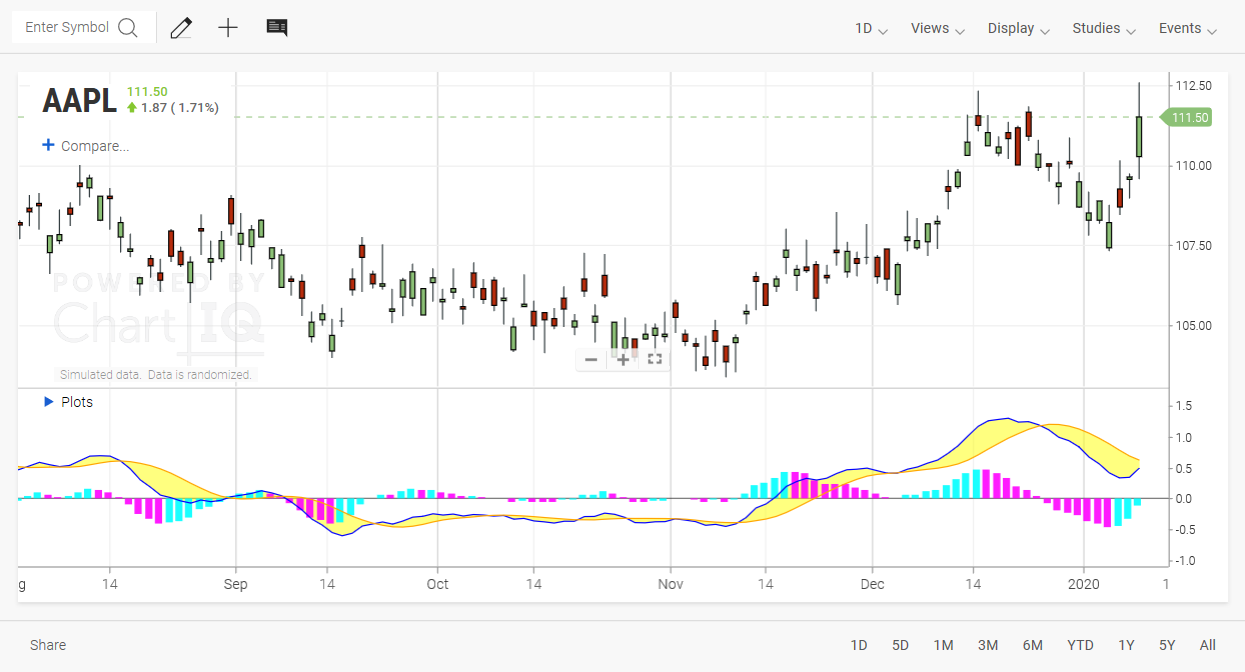Figure. Custom MACD with histogram and filled channels.

With ScriptIQ you can manipulate the data however you like and create exactly the type of display you want.

## More examples

### Simple dataset field manipulation

``````study("Custom Study")
field = input("Field", "Close")
value = dataset(field)
# Add 4 to the selected field.
plusfour = value + 4
plot(plusfour, color: "orange")
plot(value, color: "blue")
``````

### Spread between high and low

``````study("High-Low Spread")
value1 = dataset("High")
value2 = dataset("Low")
value3 = value1-value2
plot(value3, color: "white")
``````

### Standard deviation

``````study("Custom Study")
field = input("Field", "Close")
period = input("Period", 20)
value = dataset(field)
deviation = stddev(value, period)
plot(deviation)
``````

### Study overlay

``````study("Custom Overlay", overlay: true)
field = input("Field", "Close")
fast = input("Fast Period", 12)
fastMA = ema(field, fast)
plot(fastMA, color: "blue", display:"Custom")
``````

### Computing a value using the previous bar

``````study("Custom Study")
field = input("Field", "Close")
value = dataset(field)
previousBar = dataset(field, -1)
compare = value-previousBar
plot(compare)
``````